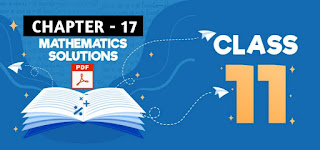# Application Of Derivatives: Class 11 Mathematics Solutions## Chapter - 17  Application Of Derivatives

In this chapter, we are basically going to learn about the methods of finding whether the given function is increasing or decreasing at certain point. We will learn to find absolute minima, absolute maxima, local maxima, local minima and point of inflection.

So it is better to have a little concept about each topic before you move to the exercises.

### Introduction

The application of derivatives plays a very important role in the field of Science engineering, economics, commerce and so on. The problems of the type "the increase in the length of an iron rod due to the increase in temperature"; "the increasing and decreasing tendency of the cost functions"; "the maximum profit to be made with the help of minim investment" and so on can betterly be solved with the help of derivatives.

### Local Maxima

A function y = f(x) is said to have the local maximum value or local maxima at x = a , if f(a) > f(a ± h) , for sufficiently small positive value 'h'.

The local maxima is known as relative maxima of the function.

### Local Minima

A function y = f(x) is said to have local minimum value at x= a if f(a) < f(a ± h), for sufficiently small positive value 'h'.

### Absolute Maxima

A function y = f(x) is said to have absolute maximum value or absolute maxima at x = a , if f(a) is greatest of all its valves for all 'x' belonging to the domain of the function.

In other word, f(a) is absolute maximum value of f(x) if f(a) ≥ f(x) for all x € D(f).

### Procedure to find the Absolute Maxima and Minima

Let y = f (x) be the given function defined in (a, b). Now,
• Find dy/dx or f'(x) i.e first derivative.
• Take f'(x) = 0, to solve for x to get stationary point. Suppose valve of x are 'c' and 'd'.
• Find the value of f(x) at x = a, b, c, d. The least value gives the absolute minimum value and greatest valve gives the absolute maximum value.

### Procedure to find Local Maxima and Local Minima

• Let y = f(x) be a function. Then,
• Find dy/dx or f'(x) and f"(x)
• Making f'(x) = 0, to solve for x to get stationary point. Let x = a be one of stationary point.
• Find f" (a).

If f"(a) < 0 then f(x) has maximum value at x=a, Maximum valve is f(a).
If f"(a) > 0 then f(x) has minimum value at x=a, Minimum value is f(a).
If f" (a) = 0 then f"'(a) ≠ 0 then f(x) has neither maximum nor minimum value.

### Exercise - 17.1

This PDF contains all the solutions of class 11, application of derivative chapter.

NoteScroll the PDF to view all Solution

You are not allowed to post this PDF in any website or social platform without permission.

## Is Class 11 Mathematics Guide Helpful For Student ?

I have published this Notes for helping students who can't solve difficult maths problems. Student should not fully depend on this note for completing all the exercises. If you totally depend on this note and simply copy as it is then it may affect your study.

Student should also use their own will power and try to solve problems themselves. You can use this mathematics guide PDF as a reference. You should check all the answers before copying because all the answers may not be correct. There may be some minor mistakes in the note, please consider those mistakes.

## How to secure good marks in Mathematics ?

As, you may know I'm also a student. Being a student is not so easy. You have to study different subjects simultaneously. From my point of view most of the student are weak in mathematics. You can take me as an example, I am also weak in mathematics. I also face problems while solving mathematics questions .

If you want to secure good marks in mathematics then you should practise them everyday. You should once revise all the exercise which are already taught in class. When you are solving maths problems, start from easy questions that you know already. If you do so then you won't get bored.

Maths is not only about practising, especially in grade 11 you to have the basic concept of the problem. When you get the main concept of the problem then you can easily any problems in which similar concept are applied.

When your teacher tries to make the concept clear by giving examples then all students tries to remember the same example but you should never do that. You can create your own formula which you won't forget later.

If you give proper time for your practise with proper technique then you can definitely score a good marks in your examination.

Disclaimer: This website is made for educational purposes. If you find any content that belongs to you then contact us through the contact form. We will remove that content from our as soon as possible.

If you have any queries then feel free to comment down.
No Comment